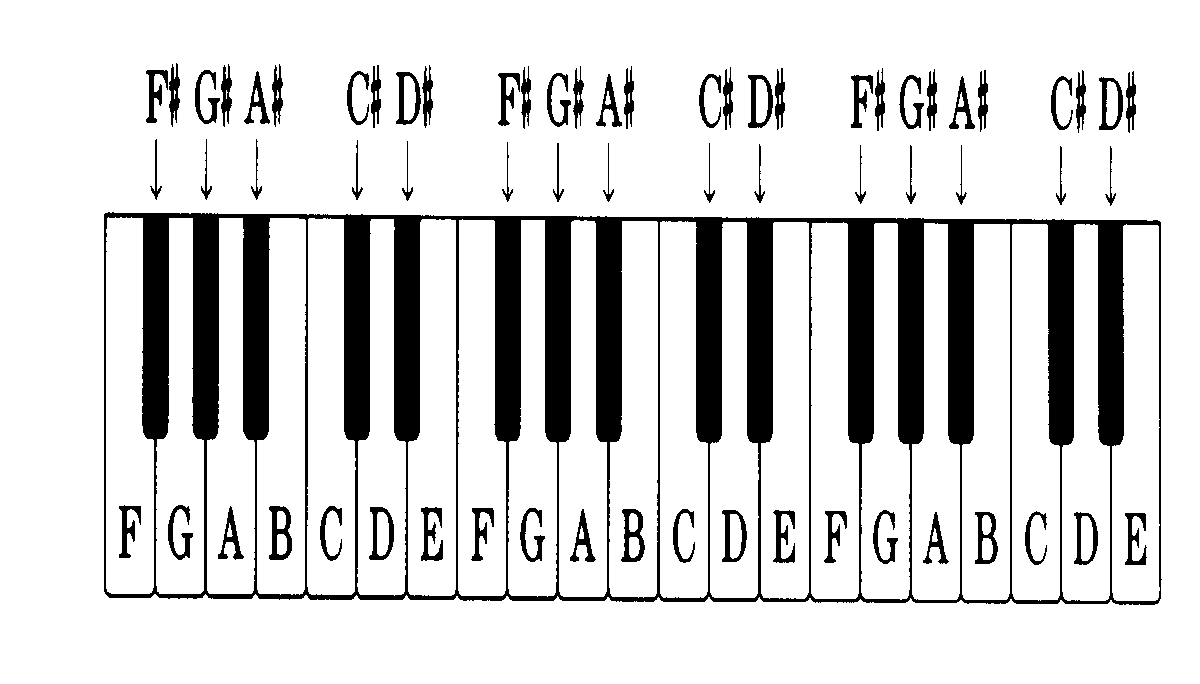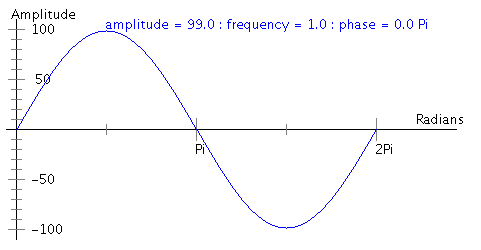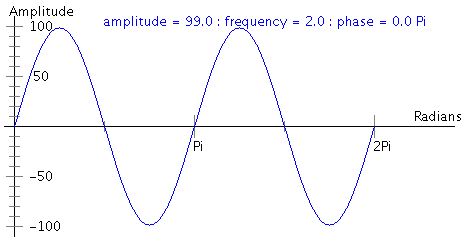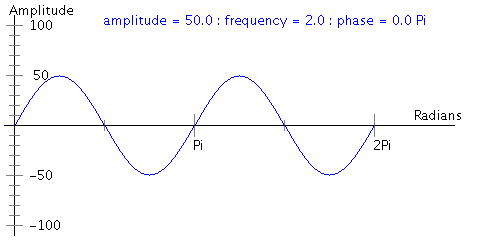# PreLab 08

The Sound of Music
Due by the beginning of lecture on Wednesday, October 29th

This week you'll be making sounds and music with Python. In this prelab, you will think about some of the underlying details of the lab itself. Please type up your solutions and hand in a printed copy before the beginning of class on Wednesday. Remember, no late prelabs allowed!

## Part 0 - Headphones

This lab is going to involve music; while some of the lab computers do have speakers, not all do, and regardless, it'll get a little crazy if everyone is trying to play sound files at the same time. So please bring a pair of headphones to lab.

## Part 1 - Music BasicsNotes are musical sounds called "pitches", that is, how high or low the note is. For example, do, re, mi are all pitches (also known as C, D, and E). Notes can be defined relative to each other; you can raise or lower the pitch by whole steps by going from one note to the next, or the pitch can be raised and lowered in half steps using sharps (#) and flats (b), respectively. These half-steps are called semitones, and there are 12 semitones in an octave (for example, the half step from C to C# is a semitone; from C# to D is as well). Each note also has a duration (how long it sounds) and a volume (how loud it sounds), so technically, a "note" is defined not only by its pitch, but also by its duration and volume.

## Part 2 - Some Physics of Music

A music note is just a sound wave. More specifically, any one note can be thought of as a sine wave, and is characterized by its [regular] frequency of oscillation (pitch), its amplitude (volume), and its length (duration). Each of these is explained below.y(t) = 99 * sin(t) for 0<=t<=2Piy(t) = 99 * sin(2t) for 0<=t<=2Piy(t) = 50 * sin(2t) for 0<=t<=2Pi

#### Frequency (Pitch)

• The frequency of a sine wave is the number of peaks per 2Pi radians. The first wave above has frequency 1, and the following two waves have frequency 2.
• The way that humans perceive pitch is associated with the frequency of the sound wave which impinges upon the ear. A high pitch sound corresponds to a high frequency sound wave (many peaks in a small span), and a low pitch corresponds to a low frequency sound wave (few peaks in a small span).
• More specifically, frequency is vibrations per second. The unit used to measure frequency is the Hertz (abbreviated Hz), where 1 Hertz = 1 vibration / second. The human ear can detect sound waves with frequencies ranging from 20Hz to 20000 Hz.
• Doubling a pitch's frequency increases the pitch by an octave, and halving it decreases it by an octave. There are 12 semitones in an octave, therefore, the ratio between the frequency of successive semitones is the 12th root of two (1.05946) (NOTE: this is not the same as 1 + 1/12). That is, to increase a pitch by a semitone, you only need to multiply its frequency by the 12th root of 2.
1) Use the above information to fill in the frequencies of the pitches below.
A A# B C C# D D# E F F# G G# A
440.00 466.16                     880.00

#### Amplitude (Volume)

• The amplitude of a sine wave is the maximum height (above 0) of any peak. The first two waves above have amplitude 99, the last wave has amplitude 50.
• The greater the amplitude, the LOUDER the sound.

#### Length (Duration)

• The length of a sine wave is, well, the length along the x-axis of the wave. The waves above have length 2Pi.
• The longer the length, the longer the duration of the sound. One second of sound corresponds to length 2Pi.

#### Multiple notes

• Each wave corresponds to a note. To play mutliple notes, you just superimpose (that is, add) the waves of each individual note. In the example below, the blue wave represents the low note, the red wave represents the note one octave higher (its frequency is double that of the blue wave), and the green wave represents both notes playing at the same time (the sum of their waves).#### Constructing Sound Waves

To construct a sound wave, you first need to know the frequency (H), the amplitude (A), and the length (T) of your wave. Once you have this information, you just need to calculate y(t) = A * sin( 2 Pi H t ) for all 0 <= t <= T. Note that t is the timestep of the note you are calculating.

Notice that y(t) is a continuous function (you can draw it without lifting your pen), and computers don't do so great with continuous stuff. Instead, the computer samples the sound wave many times per second to produce digital audio. Each sample represents the amplitude (y-value) of the sound wave at a particular instant in time (t). The sampling rate is the number of samples taken per second. Audio from CDs typically uses a sampling rate of 44100. So, instead of drawing the sound wave without lifting your pen, the computer is drawing a whole lot of dots along the same wave, with the idea that if you draw enough dots, it still looks (and sounds) like a continuous wave even though it isn't. The same idea is used in animation and those little flip books; a sequence of still frames when played at a high enough speed give the impression of smooth/continuous movement. So now if you are calculating 2 seconds of audio with a sampling rate of 44100, the values for t will be 0,1,2,3,...,88198,88199,88200.

2) If the sampling rate (R) is 44100 and the duration (T) of a note is 2.5 seconds, how many samples are needed for this note? Give a general expression for the number of samples in terms of T and R (note that the number of samples needs to be rounded to an integer).

3) Write a Python function with parameters T (length in seconds), H (frequency), A (amplitude), and R (sampling rate) that creates a list containing N samples as given by the formula y(t)=A*sin(H*2Pi*t), where N is the number calculated in the previous question. The parameters should have default values of 1, 440, 1, and 44100 respectively. Note that t will indicate the sample number - t will be 1 for the first element in the list, 2 for the second, and so on.

In the lab proper, you will be writing your own Soundwave class that will represent the discretization of a snippet of sound. Initially a particular Soundwave will hold a single digitized note, but eventually we'll want to be able to combine and overlay notes as well.

4) Given the information above, what fields (i.e. variables or attributes) should each Soundwave contain? What are the types of these fields?

5) Other than a constructor (which will probably do something similar to what you specified in your solution to question 3), what functions would it be reasonable for a Soundwave object to have? List at least 4.

## Part 3 - Making Beautiful Music

Once you've gotten a basic Soundwave class defined, much of the remainder of the lab revolves around producing music. In particular, we're going to use .wav files (a type of sound file type, much like an .mp3) to produce a variety of scales and generate a Minuet and Trio. Yes, you will write a Minuet and Trio. Sorta. But before that...

A scale is a sequence of notes, defined by the intervals between them. For example, the major scale is defined by the 7 intervals (and hence 8 notes) (2,2,1,2,2,2,1), that is, there are 2 semitones between the first and second notes, between the second and third notes, but a single semitone between the third and fourth notes, and so on. The C major scale is the major scale starting at C and is thus the sequence of notes of values starting at around 523 and ending around 1046, that is, the notes (C, D, E, F, G, A, B, C). The D major scale is the major scale starting at D: the sequence of notes (D, E, F#, G, A, B, C#, D).

6) Give the sequence of note frequencies and notes for the A major scale, starting at the A from the table from question 1 (that is, the note of frequency 440).

There are many other interesting scales, such as the minor scale, defined by the intervals (2,1,2,2,1,2,2), and the blues scale, defined by the intervals (3,2,1,1,3,2) (the scale only contains 7 notes).

7) Suppose you are given a scale, defined by its tonic (starting) note frequency F and intervals (I1, I2, I3,..., In) (that is, the second note of the scale is I1 semitones from the tonic note, the third note of the scale is I1+I2 semitones from the tonic, etc.). What is the frequency of the second note (in terms of F and I1)? What about the frequency of the third note (in terms of F, I1 and I2)?

8) Given a scale defined by its intervals (I1, I2, I3,..., In) and tonic note frequency F, give an algorithm (in pseudocode!) that produces the sequence of (n+1) note frequencies in the actual scale. That is, you have two input parameters: the start note and the sequence of intervals (in a list, say). You want to output the sequence of note frequencies in the appropriate scale.

Finally, let's talk about this Minuet and Trio business. What is a Minuet and Trio? It is musical piece that is often the third movement of the Classical sonata cycle. Both the Minuet and Trio follow a specific rhythm and form, and they are usually combined by first playing the Minuet, then playing the Trio, then the Minuet once more. You can listen to a very nice Minuet and Trio here.

You'll be generating a Minuet and Trio based on a random algorithm developed by Mr. Mozart himself. Your Minuet will contain 16 measures (musical snippets), as will your Trio. For each of the 16 measures in the Minuet, you will randomly generate a number between 0 and 10 (inclusive); you will use this number to pick a specific music snippet from the following table (there are 176 total snippets). That is, for each column in the table below, you will randomly pick a row, and use whichever measure is that (column, row) entry. For example, if I generate the 16 random numbers (1, 2, 3, 4, 5, 6, 7, 8, 9, 10, 0, 1, 2, 3, 4, 5) for the Minuet, then I will select the snippets (32, 95, 113, 45, 154, 133, 169, 123, 102, 20, 26, 56, 73, 160, 1, 151) from the Minuet table. Here is such a randomly generated Minuet and Trio. For the Trio, you do the same thing except your random number is between 0 and 5, inclusive.

Minuet Measures

0 1 2 3 4 5 6 7 8 9 10 11 12 13 14 15
0 96 22 141 41 105 122 11 30 70 121 26 9 112 49 109 14
1 32 6 128 63 146 46 134 81 117 39 126 56 174 18 116 83
2 69 95 158 13 153 55 110 24 66 139 15 132 73 58 145 79
3 40 17 113 85 161 2 159 100 90 176 7 34 67 160 52 170
4 148 74 163 45 80 97 36 107 25 143 64 125 76 136 1 93
5 104 157 27 167 154 68 118 91 138 71 150 29 101 162 23 151
6 152 60 171 53 99 133 21 127 16 155 57 175 43 168 89 172
7 119 84 114 50 140 86 169 94 120 88 48 166 51 115 72 111
8 98 142 42 156 75 129 62 123 65 77 19 82 137 38 149 8
9 3 87 165 61 135 47 147 33 102 4 31 164 144 59 173 78
10 54 130 10 103 28 37 106 5 35 20 108 92 12 124 44 131

Trio Measures

16 17 18 19 20 21 22 23 24 25 26 27 28 29 30 31
0 72 6 59 25 81 41 89 13 36 5 46 79 30 95 19 66
1 56 82 42 74 14 7 26 71 76 20 64 84 8 35 47 88
2 75 39 54 1 65 43 15 80 9 34 93 48 69 58 90 21
3 40 73 16 68 29 55 2 61 22 67 49 77 57 87 33 10
4 83 3 28 53 37 17 44 70 63 85 32 96 12 23 50 91
5 18 45 62 38 4 27 52 94 11 92 24 86 51 60 78 31

9) Write Python code that generates a Minuet and Trio by implementing the algorithm above. You may assume that the Minuet and Trio tables are stored in two-dimensional lists mTable and tTable of sizes 11x16 and 6x16, respectively. You may assume for now that there is a method concatenate that takes in a music snippet and adds it to the end of the current piece of music.

## Honor Code

If you followed the Honor Code in this assignment, write the following sentence attesting to the fact at the top of your homework.

I affirm that I have adhered to the Honor Code in this assignment.